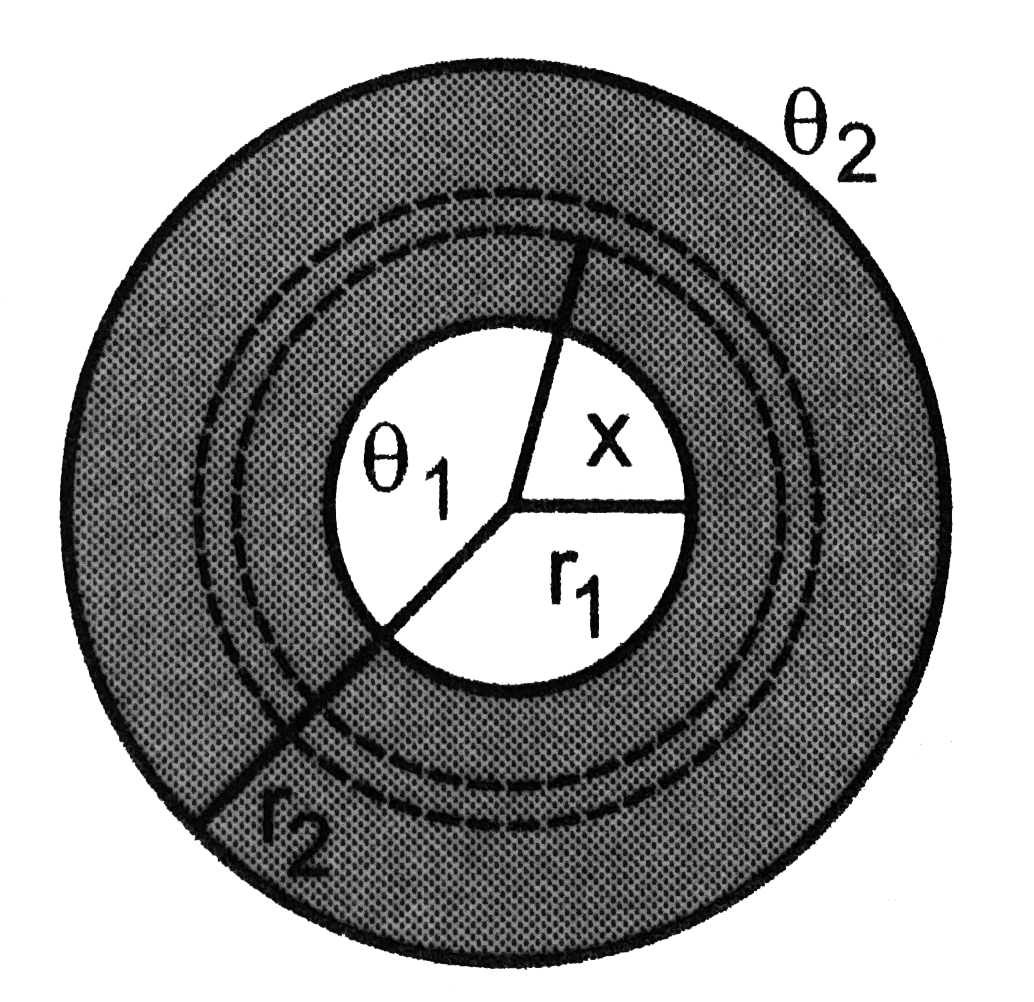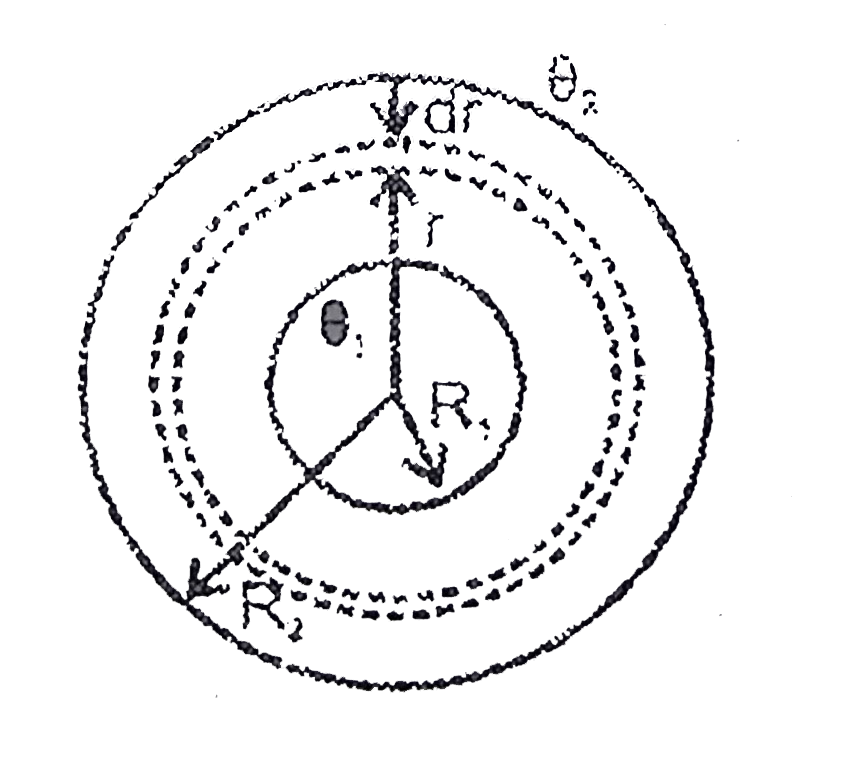# Two thin metallic spherical shells of radii r_(1) and r_(2) (r_(1)lt r_(2)) are placed with their centres coinciding. A material of thermal cond

22 views
in Physics
closed
Two thin metallic spherical shells of radii r_(1) and r_(2) (r_(1)lt r_(2)) are placed with their centres coinciding. A material of thermal conductivity K is filled in the space between the shells. The inner shells is maintained at temperature theta_(1) and the outer shell at temperature theta_(2) (theta_(1)lttheta_(2)). Calculate the rate at which heat flows radially through the material.A. (R_(1)+R_(2))/(2)
B. (R_(1)R_(2))/(R_(1)+R_(2))
C. (2R_(1)R_(2))/(R_(1)+R_(2))
D. R_(1)+(R_(2))/(2)

by (90.1k points)theta_(1) - theta_(2) = Delta theta (theta_(1)-theta)/(overset(R)underset(R_(1))int(dr)/(K4pir^(2))) =(theta_(1)-theta_(2))/(overset(R_(2))underset(R_(1))int(dr)/(K4pir^(2)))
(Delta theta//2)/((1)/(4piK_(1))[(1)/(R_(1))-(1)/(R)]) =(Delta theta)/((1)/(4piK_(1))((1)/(R_(1))-(1)/(R_(2))))
rArr R = (2R_(1)R_(2))/(R_(1)+R_(2))